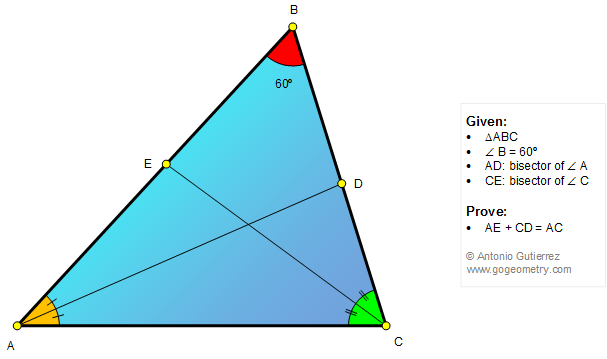# Geometry Problem 1303: Triangle, 60 Degrees, Angle Bisector, Measurement. Level: School, College, Mathematics Education

< PREVIOUS PROBLEM  |  NEXT PROBLEM >

 The figure below shows a triangle ABC with the angle B = 60 degrees. If AD and CE are the bisectors of the angles A and C, respectively, prove that AE + CD = AC.Home | Search | Geometry | Problems | All Problems | Open Problems | Visual Index | 10 Problems | Problems Art Gallery |  Art | 1301-1310 | Triangle | 60 degrees | Angle Bisector | Congruence | by Antonio Gutierrez